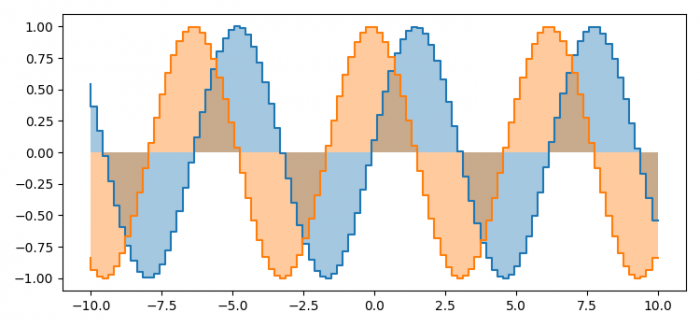# How to fill the area under a step curve using pyplot? (Matplotlib)

To fill the area under step curve using pyplot, we can take the following steps

## Steps

• Set the figure size and adjust the padding between and around the subplots.
• Ceate random data points, x, y1 and y2, using numpy.
• To fill the area under the curve, put x and y with ste="pre", using fill_between() method.
• Plot (x, y1) and (x, y2) lines using plot() method with drawstyle="steps" method.
• To display the figure, use show() method.

## Example

import matplotlib.pyplot as plt
import numpy as np

plt.rcParams["figure.figsize"] = [7.50, 3.50]
plt.rcParams["figure.autolayout"] = True

x = np.linspace(-10, 10, 100)
y1 = np.sin(x)
y2 = np.cos(x
plt.fill_between(x, y1, step="pre", alpha=0.4)
plt.fill_between(x, y2, step="pre", alpha=0.4)

plt.plot(x, y1, drawstyle="steps")
plt.plot(x, y2, drawstyle="steps")

plt.show()

## Output